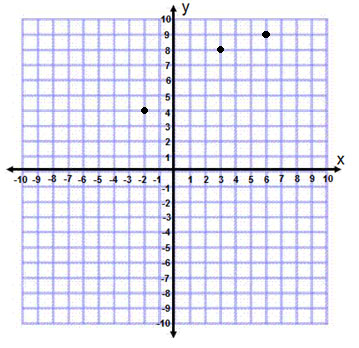# PARCC Grade 5 Math Practice Test Questions

##### 1. The number of customers in a new restaurant is given in the table below: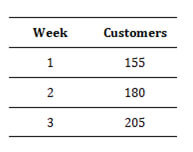1. 225
2. 230
3. 200
4. 255
##### Part B: The cost of the average meal at the restaurant is \$12. How much money should they expect to take in during week 4?

___________________________________________________

##### 2. The rectangular prism below has a volume of 144 cu. cm. The length and width are given below. What is the height of the rectangular prism?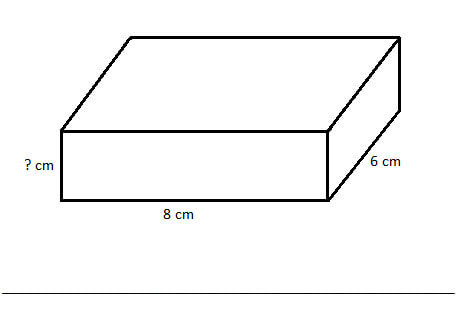##### 3. An electronics store sells E Evercell brand batteries in packages of 4 and D Durapower brand batteries in packages of 6. Which expression represents the total number of batteries in the store?
1. (4+E)x(6+D)
2. (4xE)+(6xD)
3. (4+E)+(6+D)
4. (4xE)÷(6xD)
##### 4. Using the order of operations solve the problem below.

8x(5-2)+(4+5)=_____________________

##### 5. On the graph below plot the points (6,9), (3,8), and (-2,4).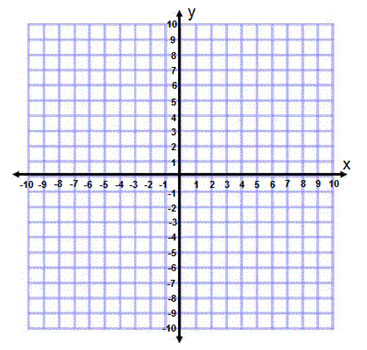1. Part A: B: The pattern in the table is that the number of customers is increasing by 25 each week. This means that there should be 205+25 =230 customers expected in week 4.

Week Customers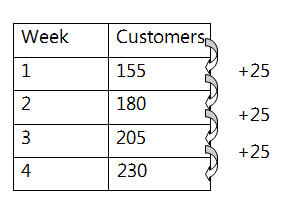Part B: \$2760: From Part A you know there will be 230 people in week 4. If each meal is \$12 then just multiply to get the answer. 230×12=2760

2. 3: The area of a rectangular prism is A=LxWxH. The problem gives L, W, and A. To get H just divide A by L and W. 144÷8=18÷6=3. So H=3.

3. B: The total number of Evercell batteries is (4xE) and the total number of Durapower batteries is (6xD). The sum of the two is the total number of batteries in the store.

4. D: The order of operations is parentheses, exponents, multiply, divide, add, subtract. So for this problem it would be: 8x(5-2)+(4+5)=8×3+9=24+9=33

5.